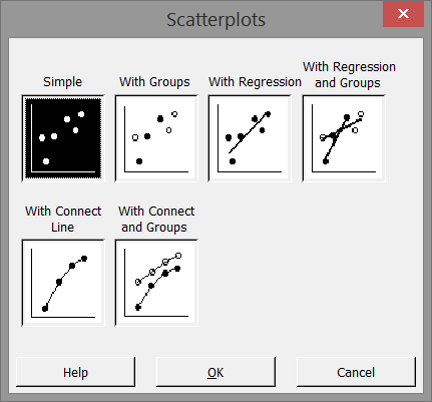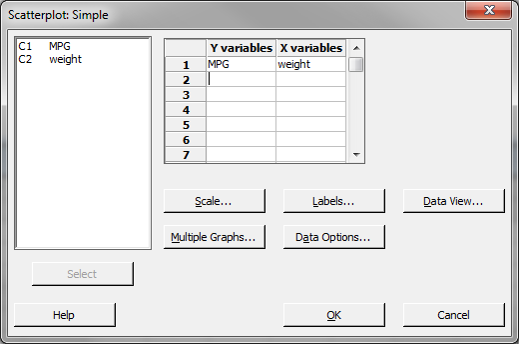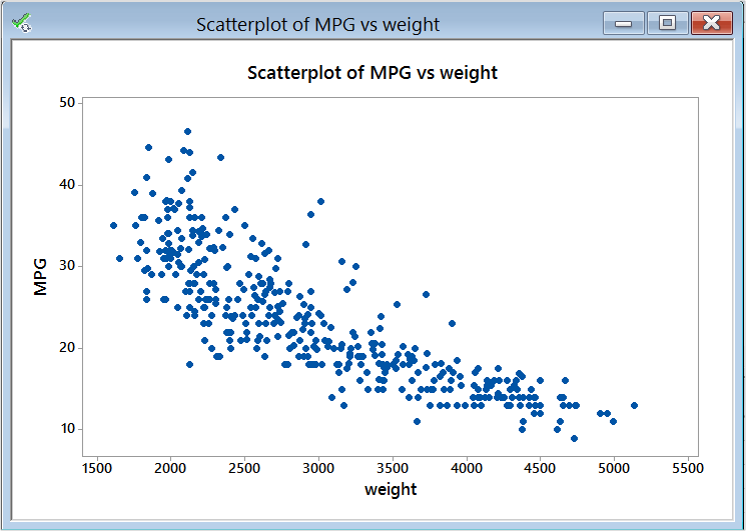# Scatter Plot with Minitab

### What is a Scatter Plot?

A scatter plot is a diagram to present the relationship between two variables of a data set. A scatter plot consists of a set of data points. On the scatter plot, a single observation is presented by a data point with its horizontal position equal to the value of one variable and its vertical position equal to the value of the other variable. A scatter plot helps us to understand:

• Whether the two variables are related to each other or not
• What the strength of their relationship
• The shape of their relationship
• The direction of their relationship
• Whether outliers are present

### How to Use Minitab to Generate a Scatterplot

Data File: “Scatter Plot” tab in “Sample Data.xlsx”

Steps to render a Scatterplot in Minitab:

1. Click Graph → Scatterplot.
2. A new window named “Scatterplots” pops up.3. Leaving “Simple” selected, click “OK”
A new window “Scatterplot– Simple” pops up.
4. Select “MPG” as the “Y variables.”
5. Select “weight” as the “X variables.”6. Click “OK.”
7. The scatter plot appears in the new window.Model summary: The figure above is Minitab’s output of the scatterplot data. You can immediately see the value of graphical displays of data. This information obtainable by viewing this output shows a relationship between weight and MPG. This scatterplot shows that the heavier the weight the lower the MPG value and vice versa.